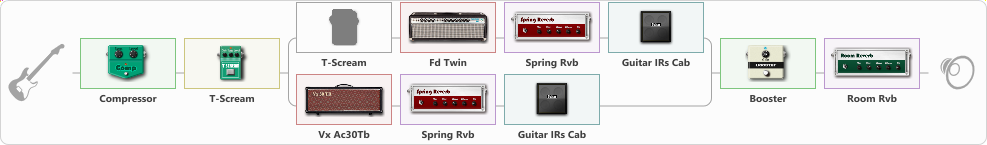# Stevie Early Tone

Discussion in 'ToneLib-GFX presets' started by josetovar, Jan 24, 2021.

1. Stevie Early Tone

Preset name: Stevie Tone

Effects chain:Effect: "Compressor" (Dynamics / Filter), active - "yes"
{
"Sense" = 21
"Level" = 50
}

Effect: "T-Scream" (Overdrive / Distortion), active - "yes"
{
"Drive" = 39
"Tone" = 71
"Level" = 60
}

Effect: "Splitter" (Dynamics / Filter), active - "yes"
{
"A-Bypass" = Off
"A-Pan" = 0
"A-Level" = 55
"B-Bypass" = Off
"B-Pan" = 0
"B-Level" = 55

'A' branch:
{

Effect: "T-Scream" (Overdrive / Distortion), active - "no"
{
"Drive" = 19
"Tone" = 53
"Level" = 79
}

Effect: "Fd Twin" (Amp simulators), active - "yes"
{
"Gain" = 51
"Bass" = 31
"Middle" = 51
"Treble" = 64
"Presence" = 80
"Master" = 70
"Level (dB)" = 0
}

Effect: "Spring Rvb" (Reverberation), active - "yes"
{
"Time" = 7.0
"PreDelay" = 0
"LoDamp" = 16
"HiDamp" = 26
"Mix" = 12
}

Effect: "Guitar IRs Cab" (Cabinets), active - "yes"
{
"Model" = Fender Deluxe Rev (1x12")
"Mic Position" = Middle
"Mic Distance" = Near
"Low Cut (Hz)" = 60
"Hi Cut (kHz)" = 20.0
"Mix" = 100
"Level (dB)" = 0
}
}
'B' branch:
{

Effect: "Vx Ac30Tb" (Amp simulators), active - "yes"
{
"Gain" = 50
"Bass" = 37
"Middle" = 75
"Treble" = 60
"Presence" = 80
"Master" = 76
"Level (dB)" = 0
}

Effect: "Spring Rvb" (Reverberation), active - "yes"
{
"Time" = 7.0
"PreDelay" = 0
"LoDamp" = 16
"HiDamp" = 26
"Mix" = 12
}

Effect: "Guitar IRs Cab" (Cabinets), active - "yes"
{
"Model" = Fender Deluxe Rev (1x12")
"Mic Position" = Middle
"Mic Distance" = Near
"Low Cut (Hz)" = 60
"Hi Cut (kHz)" = 20.0
"Mix" = 100
"Level (dB)" = 0
}
}
}

Effect: "Booster" (Dynamics / Filter), active - "yes"
{
"Gain" = 50
}

Effect: "Room Rvb" (Reverberation), active - "yes"
{
"Time" = 4.5
"PreDelay" = 0
"LoDamp" = 46
"HiDamp" = 25
"Mix" = 36
}

Note: You will need to download and install the ToneLib-GFX software to use the preset.

#### Attached Files:

• ###### Stevie_Early_Tone.tlgfx
File size:
1.1 KB
Views:
2,718
Sergejs Matvejenkovs likes this.